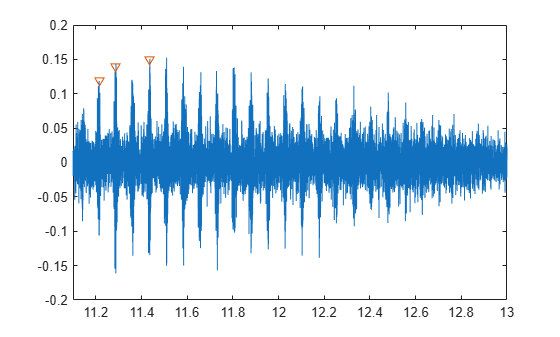Main Content

# getLabelValues

Get label values from labeled signal set

## Syntax

``val = getLabelValues(lss)``
``val = getLabelValues(lss,midx)``
``[val,sublbltbl] = getLabelValues(lss,midx,lblname)``
``[___] = getLabelValues(___,'LabelRowIndex',ridx)``
``[___] = getLabelValues(___,'SublabelRowIndex',sridx)``

## Description

example

````val = getLabelValues(lss)` returns a table containing the label values for all members of the labeled signal set `lss`.```
````val = getLabelValues(lss,midx)` returns a table containing the label values for the member specified by `midx`.```
````[val,sublbltbl] = getLabelValues(lss,midx,lblname)` returns the value of the label named `lblname`. If `lblname` has sublabels, then the table `sublbltbl` shows the structure of the label value and its sublabel variables.```
````[___] = getLabelValues(___,'LabelRowIndex',ridx)` specifies the row index, `ridx`, of an ROI or point label whose value you want to get.```
````[___] = getLabelValues(___,'SublabelRowIndex',sridx)` specifies the row index, `sridx`, of an ROI or point sublabel whose value you want to get.```

## Examples

collapse all

Load a labeled signal set containing recordings of whale songs.

```load whales lss```
```lss = labeledSignalSet with properties: Source: {2x1 cell} NumMembers: 2 TimeInformation: "sampleRate" SampleRate: 4000 Labels: [2x3 table] Description: "Characterize wave song regions" Use labelDefinitionsHierarchy to see a list of labels and sublabels. Use setLabelValue to add data to the set. ```

Get the values of the labels.

`lbls = getLabelValues(lss)`
```lbls=2×3 table WhaleType MoanRegions TrillRegions _________ ___________ ____________ Member{1} blue {3x2 table} {1x3 table} Member{2} blue {3x2 table} {1x3 table} ```

Display the moan ROI limits for the second signal of the set.

`lbb = getLabelValues(lss,2,'MoanRegions')`
```lbb=3×2 table ROILimits Value ____________ _____ 2.5 3.5 {} 5.8 8 {} 15.4 16.7 {} ```

Plot the trill region of the signal between the ROI limits. Display the labeled trill peaks.

```tvals = getLabelValues(lss,2,'TrillRegions'); peaks = getLabelValues(lss,2,{'TrillRegions','TrillPeaks'}); sg = getSignal(lss,2); plot((0:length(sg)-1)/lss.SampleRate,sg) xlim(tvals.ROILimits) hold on plot(peaks.Location,cell2mat(peaks.Value),'v') hold off```Display the coordinates of the third trill peak.

```pcoor = getLabelValues(lss,2,{'TrillRegions','TrillPeaks'}, ... 'LabelRowIndex',1,'SublabelRowIndex',3)```
```pcoor=1×2 table Location Value ________ __________ 11.437 {[0.1500]} ```

## Input Arguments

collapse all

Labeled signal set, specified as a `labeledSignalSet` object.

Example: ```labeledSignalSet({randn(100,1) randn(10,1)},signalLabelDefinition('female'))``` specifies a two-member set of random signals containing the attribute `'female'`.

Member row number, specified as a positive integer. `midx` specifies the member row number as it appears in the Labels table of a labeled signal set.

Label or sublabel name. To specify a label, use a character vector or a string scalar. To specify a sublabel, use a two-element cell array of character vectors or a two-element string array:

• The first element is the name of the parent label.

• The second element is the name of the sublabel.

Example: `signalLabelDefinition("Asleep",'LabelType','roi')` specifies a label of name `"Asleep"` for a region of a signal in which a patient is asleep during a clinical trial.

Example: `{'Asleep' 'REM'}` or `["Asleep" "REM"]` specifies a region of a signal in which a patient undergoes REM sleep.

Label row index, specified as a positive integer. This argument applies only for ROI and point labels.

Sublabel row index, specified as a positive integer. This argument applies only when a label and sublabel pair has been specified in `lblname` and the sublabel is of type ROI or point.

## Output Arguments

collapse all

Label values, returned as a table.

Sublabel values, returned as a table showing the structure of the label value and its sublabel variables.

• If `lblname` has no sublabels, then `sublbltbl` is empty.

• If you specify `lblname` as a string or cell array, then `sublbltbl` is empty.

## See Also

Introduced in R2018b

## SupportGet trial now